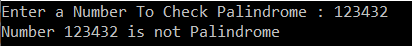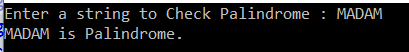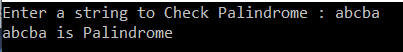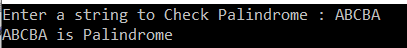# Palindrome Program in C#

## Palindrome Program (Number and String) in C# with Examples

In this article, I will discuss the Palindrome Program in C# (Palindrome Number and Palindrome String) with Examples. Please read our previous article, discussing the Prime Number Program in C# with Examples. This is one of the interview questions asked in the interview to write the logic to check whether a given number or string is palindrome or not. As part of this article, we will discuss the following pointers.

1. What is Palindrome?
2. How to check if a given number is Palindrome or not?
3. How to check if a given string is Palindrome or not?
##### Palindrome Number:

A palindrome is a word, phrase, number, or other sequence of characters that reads the same forward and backward (ignoring spaces, punctuation, and capitalization). For example, a Palindrome number is a number that is going to be the same after reversing the digits of that number. For example, numbers such as 121, 232, 12344321, 34543, 98789, etc. are palindrome numbers.

##### How to check if a given number is Palindrome or not in C#?

Algorithm to check Palindrome Number in C#:

1. First, get the number from the user which you want to check
2. Hold that number in a temporary variable
3. Reverse that number
4. Compare the temporary number with the reversed number
5. If both numbers are the same, then print it as a palindrome number else, print it as not a palindrome number
##### Example to Understabd Palindrome Number Program in C#

The following C# Program will allow the user to input a number and then check whether that number is a Palindrome Number or not.

```using System;
namespace LogicalPrograms
{
public class Program
{
static void Main(string[] args)
{
Console.Write("Enter a Number To Check Palindrome : ");
int remineder, sum = 0;
int temp = number;
while (number > 0)
{
//Get the remainder by dividing the number with 10
remineder = number % 10;

//multiply the sum with 10 and then add the remainder
sum = (sum * 10) + remineder;

//Get the quotient by dividing the number with 10
number = number / 10;
}
if (temp == sum)
{
Console.WriteLine(\$"Number {temp} is Palindrome.");
}
else
{
Console.WriteLine(\$"Number {temp} is not Palindrome");
}
}
}
}
```
###### Output:##### How to Check if a given String is Palindrome or not in C#?

In the following program, we take the string as input from the console. Then, we reverse the string using a for loop and storing the reverse string value in the reverse variable. Finally, we check whether the original and reverse values are the same or not. If both are the same, then the string is Palindrome else, it is not Palindrome.

```using System;
namespace LogicalPrograms
{
public class Program
{
static void Main(string[] args)
{
Console.Write("Enter a string to Check Palindrome : ");
string reverse = string.Empty;

for (int i = name.Length - 1; i >= 0; i--)
{
reverse += name[i];
}

if (name == reverse)
{
Console.WriteLine(\$"{name} is Palindrome.");
}
else
{
Console.WriteLine(\$"{name} is not Palindrome");
}
}
}
}
```
###### Output:##### Using the for-each loop:

Let us see how to do the previous program using a for each loop.

```using System;
namespace LogicalPrograms
{
public class Program
{
static void Main()
{
Console.Write("Enter a string to Check Palindrome : ");
string reverse = string.Empty;
foreach (char c in name)
{
reverse = c + reverse;
}
if (name.Equals(reverse, StringComparison.OrdinalIgnoreCase))
{
Console.WriteLine(\$"{name} is Palindrome");
}
else
{
Console.WriteLine(\$"{name} is not Palindrome");
}
}
}
}```
###### Output:##### Another Approach to Implementing Palindrome String Program in C#:

In the following example, first, we convert the string to a character array. Then, using the Array class Reverse method, we are reversing the elements of the character array. Once we reverse the elements of the character array, then we create a string from this array. Finally, we compare this newly created string with the original string and print it is Palindrome if both the strings are the same, else we are print it as not Palindrome.

```using System;
namespace LogicalPrograms
{
public class Program
{
static void Main()
{
Console.Write("Enter a string to Check Palindrome : ");

char[] nameArray = name.ToCharArray();
Array.Reverse(nameArray);
string reverse = new string(nameArray);

if (name.Equals(reverse, StringComparison.OrdinalIgnoreCase))
{
Console.WriteLine(\$"{name} is Palindrome");
}
else
{
Console.WriteLine(\$"{name} is not Palindrome");
}
}
}
}```
###### Output:Here, in this article, I try to explain the different ways to check whether a number or a string is Palindrome or not using C#, and I hope you enjoy this Palindrome Program in C# with Examples article. In the next article, I will discuss how to reverse a number and a string in C# with some examples.

### 3 thoughts on “Palindrome Program in C#”

1.why do you use the -1 in the for loop in the string example
i don’t really get that. can you please explain

1.I’m guessing you already knew this, but for anyone who doesn’t, the length of a string or array is not the same as the string or array’s last index value.

Meaning:

Assume you have a string text “241253.”

The text is 6 characters long.

The index position of 6 does not, however, exist. This is due to the fact that indexes begin counting at zero. That means, the text’s last index is 5.

Hence the use of Length – 1, i.e. 6 – 1. We now have 5.

So we look for text if we need the last index. The final element is 3 as a result of this.

Does that make sense?